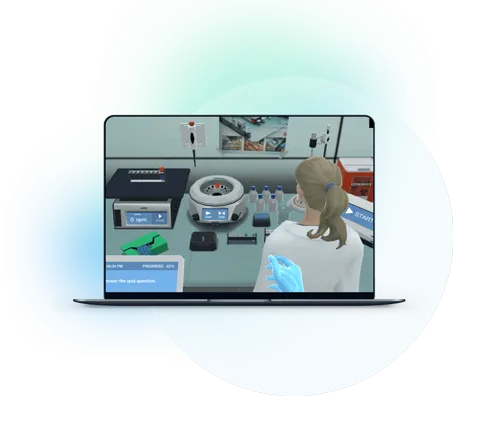Blog

# 5 Engaging Methods to Teach Chemical Formulas and Equation Balancing

Anjaney Kothari
May 26, 2023

5 Engaging Methods to Teach Chemical Formulas and Equation Balancing

Some chemistry students find chemical formulas and equation balancing so complicated that they dread learning about these topics. Others consider these topics too trivial to take seriously. But formulas and equation balancing are crucial, basic topics that can significantly impact the functioning of many laboratories and industries.

There is an undeniable need to find teaching methods that make chemical formulas and equation balancing more student-friendly. Here, we list five engaging methods to teach chemical formulas and equation balancing that will make these topics approachable for students.

## 1. Engage Students with Interactive Models

Chemistry lessons can become really tough really fast if students do not get to visualize chemical structures in some way. Interactive 3D models can keep students engaged with the subject matter, while making it easier and approachable. This is particularly true when teaching chemical formulas and equation balancing.

For example, you can try explaining the stoichiometry of chemical reactions with the help of ball-and-stick models of chemical compounds. This way, the students get to touch tangible ‘reactant molecules’ and reorganize them into the right reaction products.

## 2. Make the Topic Fun with Games and Activities

Games and activities energize students and infuse into them the fervor to learn even the most challenging topics. Plus, gamified learning environments give the students the much-needed scope of making mistakes and learning from them.

For example, in Labster’s ‘formulas and equation balancing’ virtual lab, students compete against time to balance chemical equations. With each chemical equation they balance, they get one step closer to protecting the planet from a devastating comet. This level-by-level, gamified learning gives students a chance to learn from their mistakes and have fun along the way.

## 3. Accelerate Learning by Incorporating Technology

Technologies, like simulation, animation, and interactive virtual reality, are changing the way we teach and learn about chemical structures. They have the capability to bring invisible molecules to life, so students can better grasp chemical formulas and reactions.

Take Labster’s ‘formulas and equation balancing’ virtual lab, for example. This simulation allows students to work with virtual 3D ball-and-stick models of different chemical compounds. The students can put the virtual molecules on a weighing scale to learn the law of conservation of mass. They also get to balance different chemical equations interactively. When they balance an equation correctly, the virtual lab performs the reaction for them to use later in the simulation.

Discover Labster's Formulas and Equation Balancing virtual lab today!

## 4. Inspire Students Through Career Exploration

Imagine your students learning chemical formulas and equation balancing on a whiteboard in a classroom. Here is what many of these students are asking themselves:

·   “Why do I need to learn this?”

·   “Can chemical formulas and equation balancing help me build a career?”

You can inspire your students to learn challenging as well as seemingly trivial topics by answering the above questions.

Have an interactive discussion with your students about which professions require an extensive knowledge of chemical formulas and equation balancing. Talk to them about how analytical chemists use chemical formulas and equation balancing to determine the concentrations of relevant substances. Tell them how biochemists setting up a colorimetric reaction to assay for a metabolite need to know the chemical equation and the quantity of the reagents to add for the reaction to reach completion.

## 5. Connect Topic to Real-World Applications

Chemical formulas and equations may seem trivial to some students, especially if they consider them to be mere theoretical concepts. Providing a background on the real-world applications of these topics will inspire them to take formulas and equation balancing seriously.

Here are some real-world applications of chemical formulas and equation balancing that you could share with your students:

·   Synthesis of a life-saving drug necessarily requires chemists to know the related chemical formulas and equations.

·   Energy production reactions, including nuclear and fuel cell reactions depend on the knowledge of chemical formulas and equation balancing.

## Final thoughts

To encourage students to learn chemical formulas and equation balancing, educators must employ creative tactics that engage the students while informing them about the necessity of this topic. The methods we have discussed here will allow you to make chemical formulas and equation balancing an approachable topic.

Try our free 30-day All Access Educator's Pass today and teach with the Chemical Formulas and Equation Balancing simulation alongside 300+ other virtual labs!What exactly are virtual labs?